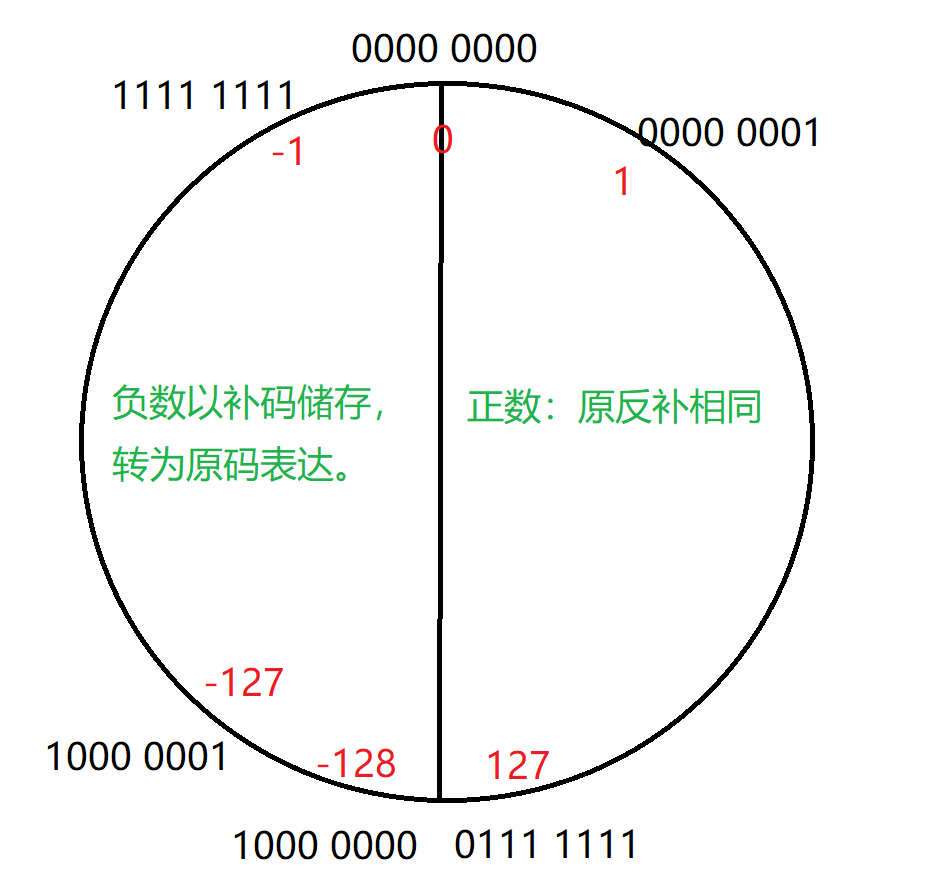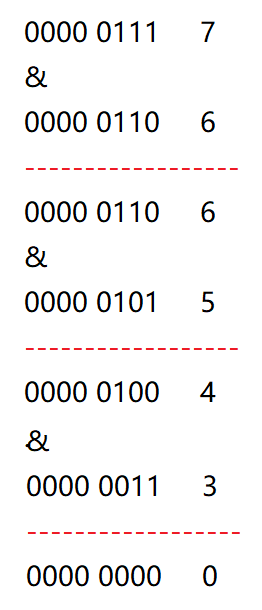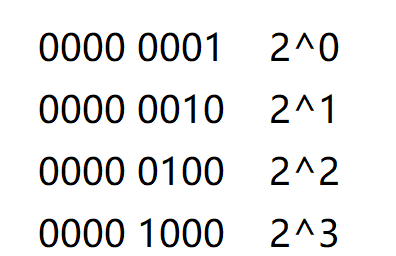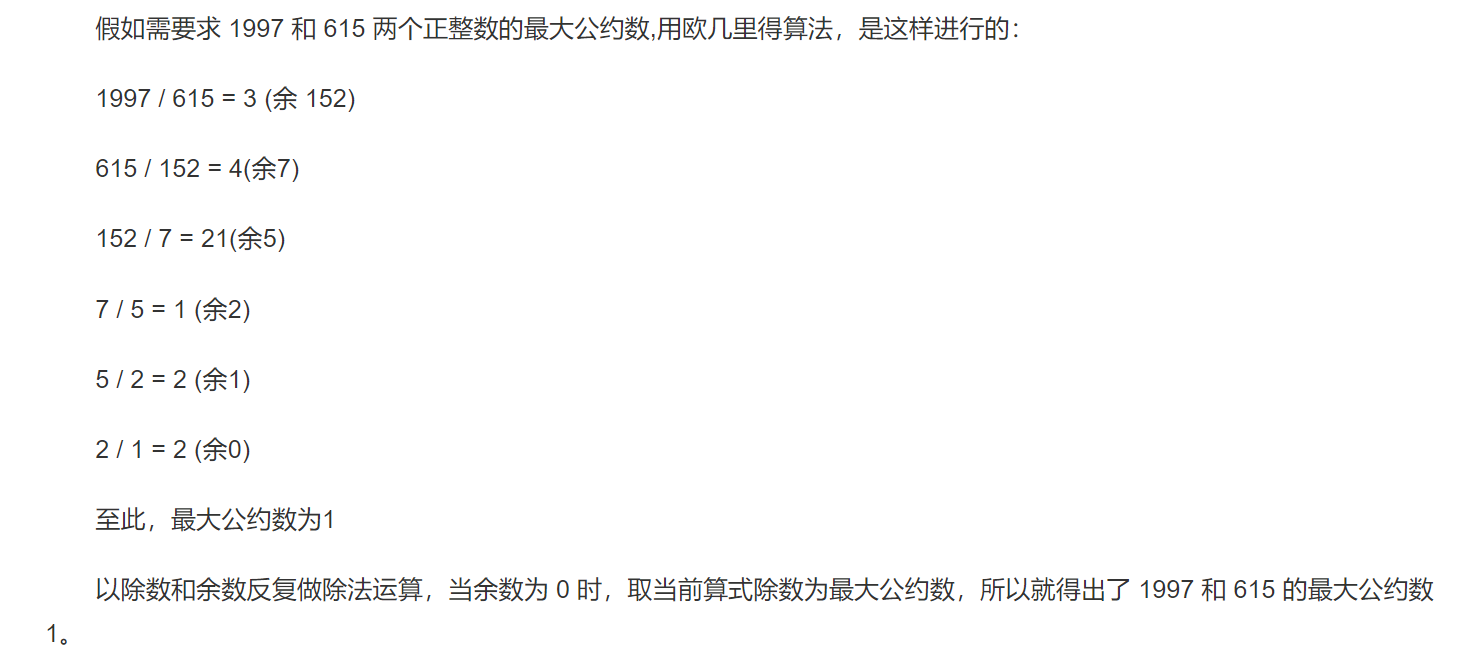0

# JavaSE(5)-九千字Java基础知识典例の深入综合解析

### 选择题

T1：Java 的字符类型采用的是 Unicode编码方案，每个 Unicode码占用（ ）个比特位

A.8 B.16 C.32 D.64

1Byte = 8个二进制位

1Byte = 2个十六进制

65535的二进制表达为1111 1111 1111 1111

T2：下列语句哪些是正确的 ( )

A.Java程序经编译后会产生machine code

B.Java程序经编译后会产生byte code

C.Java程序编译使用命令javac，运行则使用 java 命令

D.以上都不正确

``````CPU可直接解读
``````

byte code是字节码，字节码是一种中间状态的二进制代码，它比机器码更抽象，需要通过直译器转译后才能翻译为机器码。字节码的实现方式是通过编译器和虚拟机器。编译器将源码编译成字节码，特定平台上的虚拟机器将字节码转译为可以直接执行的指令。字节码在运行时通过

``````JVM（JAVA虚拟机）
``````

T3：下列有关JDK, JRE, JVM三者的叙述，正确的是：（ ）

A.JDK是Java开发工具包 ，包括了Java运行环境JRE（Java Runtime Envirnment）、一堆Java工具（javac/java/jdb等）和Java基础的类库（即Java API 包括rt.jar）。

B.JRE是运行基于Java语言编写的程序所不可缺少的运行环境。也是通过它，Java的开发者才得以将自己开发的程序发布到用户手中，让用户使用。

C.JVM是整个Java实现跨平台的最核心的部分，所有的Java程序会首先被编译为.class的类文件，这种类文件可以在虚拟机上执行。也就是说class并不直接与机器的操作系统相对应，而是经过虚拟机间接与操作系统交互，由虚拟机将程序解释给本地系统执行

D.JDK中包含了JRE,JRE又包含JVM

T4：下面属于java基本类型的有（ ）

A.String B.byte C.char D.Array

T5.下面属于java包装类的是（ ）

A.String B.Long C.Character D.Short

T6.在java中，下列标识符不合法的有（ ）

A.new B.\$Usdollars C.1234 D.car.taxi

A是关键字，错误。C以数字开头，错误。D有小数点，不符合规定，错误。

T7.经过强制类型转换以后，变量a，b的值分别为多少（ ）

``````short a =128；
byte b =(byte) a；
``````

A.128，127 B.128，-128 C.128，128 D.编译错误

128显然在short的范围内，所以可以存入a，所以a=128。

128超出了byte的范围，所以看作4字节的int类型，32位bit

``````128的原码：0000 0000 0000 0000 0000 0000 1000 0000
``````
``````128的反码：0111 1111 1111 1111 1111 1111 0111 1111
``````
``````128的补码：0111 1111 1111 1111 1111 1111 1000 0000
``````T7.如下Java语句 double x=2.0; int y=4; x/=++y; 执行后，x的值是（ ）

A.0.5 B.2.5 C.0.4 D.2.0

T8.下列说法正确的是（ ）

A.布尔类型boolean占有一个字节，由于其本身所代码的特殊含义，boolean类型与其他基本类型不能进行类型的转换（既不能进行自动类型的提升，也不能强制类型转换）， 否则，将编译出错。

B.byte a = 130; float b = 3.5; 这两行代码会发生编译错误

C.int a = 3; byte b = a; 这句话可以正常通过编译，且运行不会发生错误。

• 1个bit：理由是boolean类型的值只有true和false两种逻辑值，在编译后会使用1和0来表示，这两个数在内存中只需要1位（bit）即可存储。位是计算机最小的存储单位。
• 1个字节:理由是虽然编译后1和0只需占用1位空间，但计算机处理数据的最小单位是1个字节，1个字节等于8位，实际存储的空间是：用1个字节的最低位存储，其他7位用0填补，如果值是true的话则存储的二进制为：0000 0001，如果是false的话则存储的二进制为：0000 0000
• 4个字节：理由来源是《Java虚拟机规范》一书中的描述：“虽然定义了boolean这种数据类型，但是只对它提供了非常有限的支持。在Java虚拟机中没有任何供boolean值专用的字节码指令，Java语言表达式所操作的boolean值，在编译之后都使用Java虚拟机中的int数据类型来代替，而boolean数组将会被编码成Java虚拟机的byte数组，每个元素boolean元素占8位”。这样我们可以得出boolean类型占了单独使用是4个字节，在数组中又是1个字节。

T9.基本数据类型均可任意互相转换（ ）

A.对 B.错

T10.如果要打印一个字符串,需要使其输出结果为："hello"，则以下哪种方式可以正确输出：( )

A.System.out.println("\"hello\"");

B.System.out.println("\"hello\"");

C.System.out.println("/"hello/"");

T11.以下会产生信息丢失的类型转换是( ）

A.float a=10; B.int a =（int）8846.0; C.byte a=10;int b=-a; D.double d=100;

B，范围大的double被强转为int，丢掉了小数点后的位，信息发生丢失。

C，当操作数是byte,short,char时，它们参与运算，会自动转化为int类型，byte类型的a加上-号，参与了运算，int类型存入int类型，完全可行。

D，int类型存入double类型，范围小的数据存入范围大的数据，发生隐式（自动）类型转换，完全可行。

T12.以下哪一个不是赋值符号( )

A.+= B.<<= C.<<<= D.>>>=

T13.switch（）{}结构中，括号中可使用的数据类型不能是（ ）。

A.long B.int C.short D.float

### 编程题

T1.输出n*n的乘法口诀表，n由用户输入。

``````public static void Multiplication(int n){
int i=0;
int j=0;
for(i=1;i<=n;i++) {
for (j=1;j<=i;j++) {
System.out.print(j+"*"+i+"="+i*j+"\t");
}
System.out.println("\n");
}
}
public static void main(String[] args) {
Scanner scan = new Scanner(System.in);
System.out.println("请输入n=>");
int n= scan.nextInt();
Multiplication(n);
}
``````

T2. 编写代码模拟三次密码输入的场景。 最多能输入三次密码，密码正确，提示“登录成功”,密码错误， 可以重新输 入，最多输入三次。三次均错，则提示退出程序

``````  public static void login() {
int count = 3;
Scanner scanner = new Scanner(System.in);
while (count != 0) {
System.out.println("请输入你的密码：");
System.out.println("登录成功！");
return;
}else{
count--;
System.out.println("你还有 "+count+" 次机会！");
}
}
}
``````

==比较两个变量本身的值，即两个对象在内存中的首地址。

equals()比较字符串中所包含的内容是否相同

T3.求一个整数，在内存当中存储时，二进制1的个数。

``````  public static void main(String[] args) {
Scanner scan =new Scanner(System.in);
int i;
int count=0;//计数器
System.out.println("请输入n=>");
int n=scan.nextInt();
for(i=0;i<=31;i++){
if(((n>>i)&1)==1){
count++;
}
}
System.out.println(n+"的二进制中有"+count+"个1");
}
``````

``````public static void main(String[] args) {
Scanner scan =new Scanner(System.in);
int count=0;
System.out.println("请输入n=>");
int n=scan.nextInt();
int temp=n;
while(n!=0){
if((n&1)==1){
count++;
}
n>>>=1;
}
System.out.println(temp+"的二进制中有"+count+"个1");
}
````````````public static void main(String[] args) {
Scanner scan =new Scanner(System.in);
int count=0;
System.out.println("请输入n=>");
int n=scan.nextInt();
int temp=n;
while(n!=0){
n=(n&n-1);
count++;
}
System.out.println(temp+"的二进制中有"+count+"个1");
}
``````

T4. 获取一个数二进制序列中所有的偶数位和奇数位， 分别输出二进制序列

``````public static void main4(String[] args) {
Scanner scan =new Scanner(System.in);
int i;
System.out.println("请输入n=>");
int n=scan.nextInt();
System.out.println("偶数位:");
for(i=31;i>=1;i-=2){//打印偶数位
System.out.print((n>>>i)&1);
}
System.out.println("\n奇数位：");
for(i=30;i>=0;i-=2){//打印奇数位
System.out.print((n>>>i)&1);
}
}
``````

T5.输入一个数字k判断它是不是2的n次方`````` public static void main(String[] args) {
Scanner scan =new Scanner(System.in);
int k= scan.nextInt();
if((k&(k-1)) ==0){
System.out.println("YES");;
}else{
System.out.println("NO");
}
}
``````

T5. 求两个正整数的最大公约数

``````public static void main5(String[] args) {
Scanner scan= new Scanner(System.in);
System.out.println("请输入a，b的值=>");
int a= scan.nextInt();
int b= scan.nextInt();
int min=a>b?b:a;
for (int i = min; i >= 0 ; i--) {
if(a%i==0&&b%i==0){
System.out.println(i+"是最大公约数");
break;
}
}
}
```````````` public static void main(String[] args) {
Scanner scan= new Scanner(System.in);
System.out.println("请输入a，b的值=>");
int a= scan.nextInt();
int b= scan.nextInt();
int extra =a%b;
while(extra!=0){
a=b;
b=extra;
extra=a%b;
}
System.out.println("最大公约数为"+b);
}
``````

``````public static int gcd(int m,int n) {
if(n == 0){
return m;
}
int r = m%n;
return gcd(n,r);
}

public static void main(String[] args) {
Scanner scan= new Scanner(System.in);
System.out.println("请输入a，b的值=>");
int a= scan.nextInt();
int b= scan.nextInt();
int max=gcd(a,b);
System.out.println("最大公约数为"+max);
}
``````

T6.求出0～999999之间的所有“自幂数”并输出。(“自幂数”是指一个n位数，其各位数字的n次方和确好等于该数本身，如；153＝1^3＋5^3＋3^3，则153是一个“自幂数“。）

``````   public static void main(String[] args) {
for (int num = 0; num <=999_999; num++) {
int count=0;//位数
int temp=num;
int sum=0;//累加器
while(temp!=0){
temp/=10;
count++;
}
temp=num;
while(temp!=0){
sum+=Math.pow(temp%10,count);//+=具有自动转换的作用，pow的返回值本来是double，但是被+=强转为了int
temp/=10;
}
if(sum==num){
System.out.println(num);
}
}
}
``````

T7.判断一个数是不是素数

`````` public static boolean isPrime1(int num) {
for (int i = 2; i < num; i++) {
if(num % i == 0) {
//System.out.println("不是素数！");
return false;
}
}
//System.out.println("是素数！");
return true;
}
``````

116,28,44,82,16*1,把1的情况排除掉，发现参与运算最大数也就是16的一半8，于是可以优化算法，直接折半：

`````` public static boolean isPrime2(int num) {
for (int i = 2; i <= num/2; i++) {
if(num % i == 0) {
return false;
}
}
return true;
}
``````

``````  public static boolean isPrime2(int num) {
for (int i = 2; i <= Math.sqrt(num); i++) {
if(num % i == 0) {
return false;
}
}
return true;
}
``````

T8.判断输入的年份是不是闰年(要求多组输入！)

`````` public static void main(String[] args) {
int year=0;
Scanner scan=new Scanner(System.in);
while(scan.hasNextInt()){
System.out.println("请输入year=>");
year=scan.nextInt();
if(year%100!=0&&year%4==0||year%400==0){
System.out.println("YES");
}else{
System.out.println("NO");
}
}
}
``````

### “JavaSE(5)-九千字Java基础知识典例の深入综合解析”的评论:

##### 关于作者##### overfit同步小助手